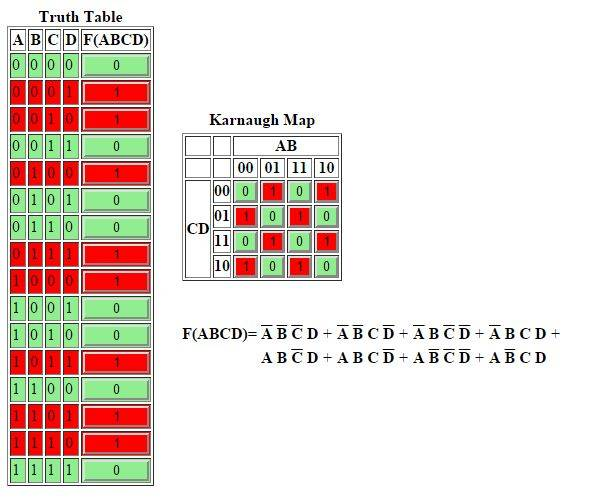# XOR gate circuits

• Engineering
Z(A,B,C,D) = SUM-M(1,2,4,7,8,11,13,14)

Draw circuit of Z with lowest number of 2 inputs xor-gates.

Can i have a clue? I tried drawing k map and had too many terms.

I tried drawing k map and had too many terms.
Can you show us what you've done?So from the K map, in the 1st row, AB looks like a xor.

•Greg Bernhardt
bump.!

gneill
Mentor
Your observation about the first row of the map is good. Note how the third row is identical. Note also how the other rows are the negation of them. Contemplate how you might build a NOT gate from an XOR. Does it give you any ideas?

Thanks for tip!

Ok so With a xor gate i can get a'b+ ab' as an output. Lets call this output as P.

Next step is for me to get c'd' + cd which is simply xnor? So i will use a xor(c,d)=Q. Th3n ill xor(Q,1) to get the inverse?

Now to combine both P and Q do i need to construct AND gate with xor?

gneill
Mentor
Thanks for tip!

Ok so With a xor gate i can get a'b+ ab' as an output. Lets call this output as P.
Okay, fine. That's a XOR b. It'll come in handy.
Next step is for me to get c'd' + cd which is simply xnor? So i will use a xor(c,d)=Q. Th3n ill xor(Q,1) to get the inverse?

Now to combine both P and Q do i need to construct AND gate with xor?
It's getting complicated again. If I may suggest a short digression:

Did you spend any time playing with the XOR gate to try to make a NOT gate? Hint: you can assume access to constants TRUE and FALSE (or 1 and 0) to use as inputs anywhere you like. If you feed one input of an XOR with a fixed 0, what does it do to the other input? How about if you use a fixed 1? Can you think of any interesting uses for such a beast? How about if the "fixed" input were actually another signal?

Hi! In my reply i used a fixed "1". Xor(Q, 1)

gneill
Mentor
Hi! In my reply i used a fixed "1". Xor(Q, 1)
Okay, but maybe you can think of another signal to use for the "1" that would select the appropriate action for that XOR to match the other rows in the table? What values of cd are associated with the "straight" a XOR b, and which with its complement?

•kukumaluboy
Challenge acceptedHi my finam answeer

Q = XOR(A , B)
P = XOR(C , D)
ANS = XOR(Q , P)

NascentOxygen
Staff Emeritus
Hi my finam answeer

Q = XOR(A , B)
P = XOR(C , D)
ANS = XOR(Q , P)
That seems to work.

gneill
Mentor
Hi my finam answeer